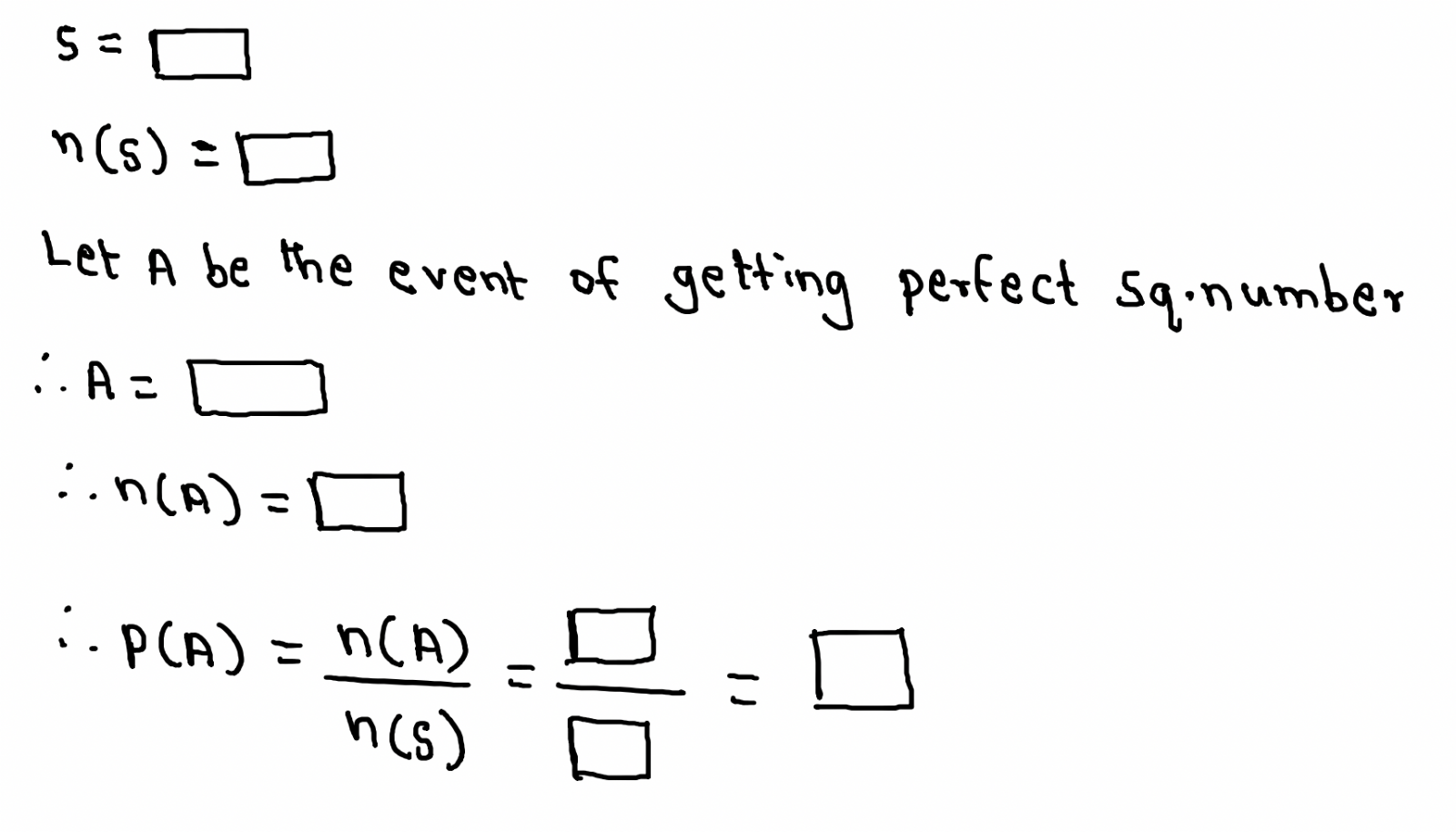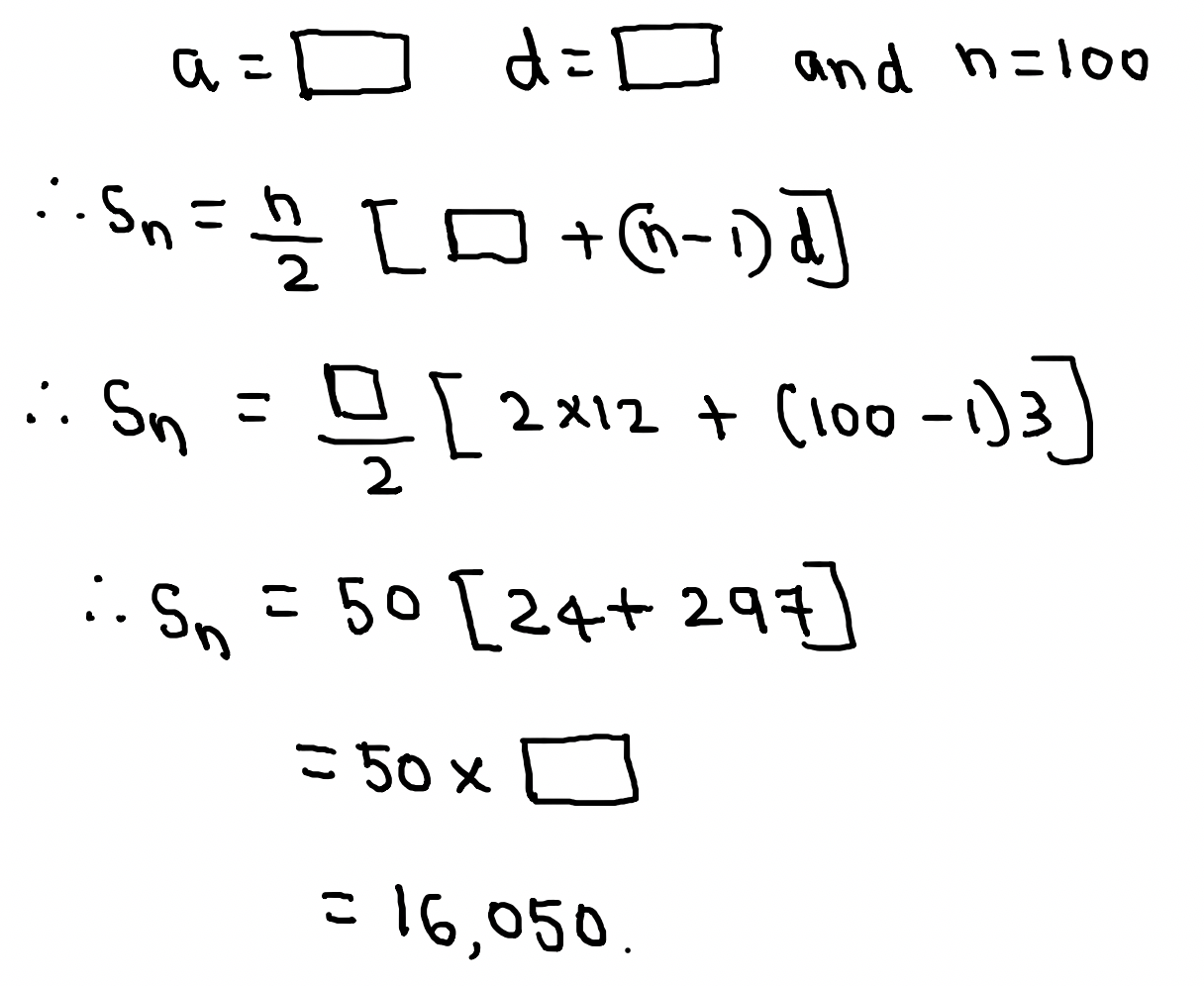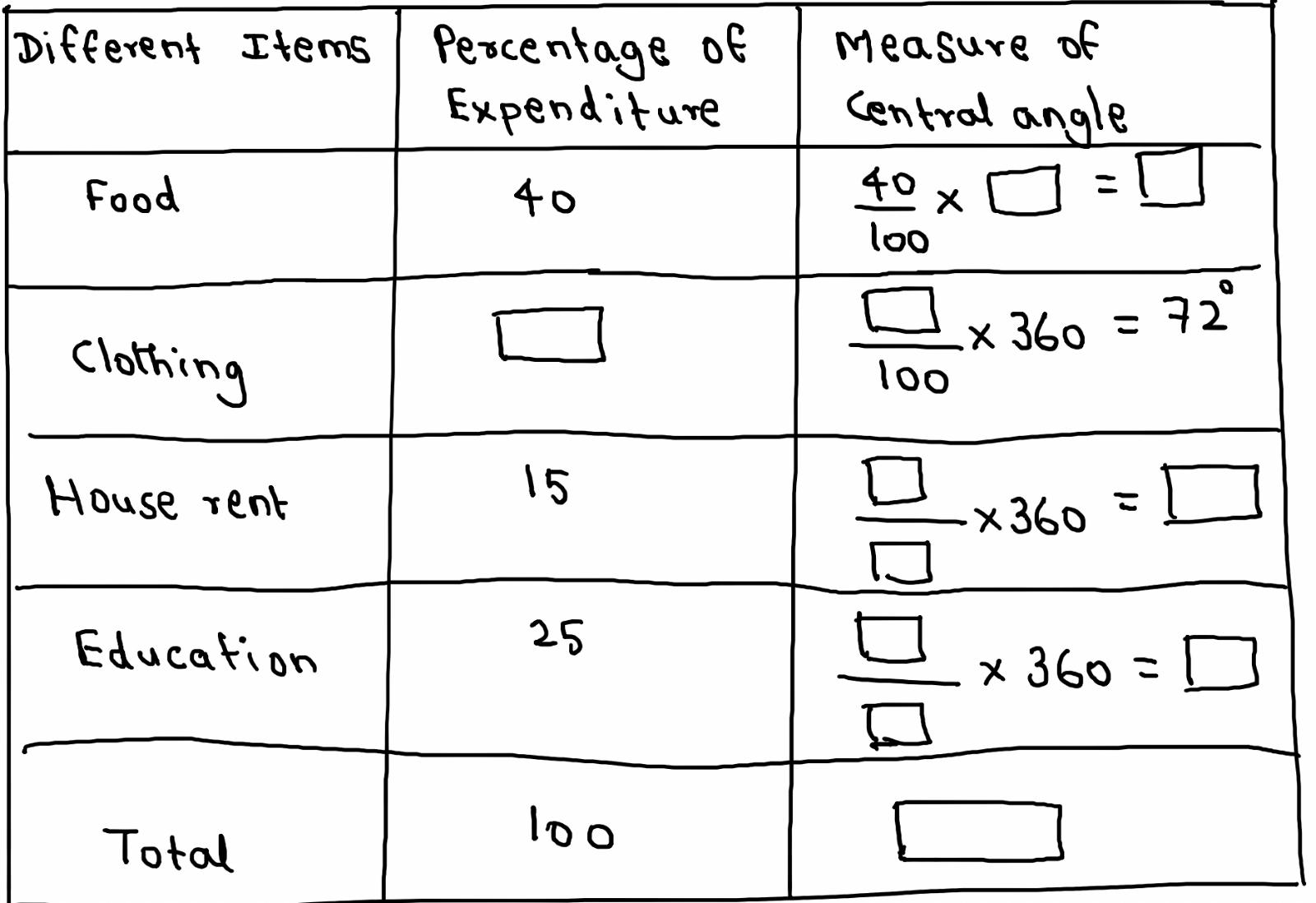### Mathematics Part – I Paper Pattern and Guidelines

Mathematics Part – I Paper Pattern and Guidelines
 Written Examination: Mathematics (Part - I) 40 Marks Time 2 Hours. Mathematics (Part - II) 40 Marks Time 2 Hours. Internal Assessment 20 Marks Total 100 Marks.

Format of the Activity Sheet.
 Marks Marks with Option Q. 1. (A) Solve any 4 out of 6 sub questions (1 marks each) 4 6 (B) Solve any 2 out of 3 sub questions (2 Marks Each) 4 6 Q. 2. (A) Solve 4 out of 4 MCQ (1 Mark Each) 4 4 (B) Solve 2 out of 3 subquestions. (2 Marks Each) 4 6 Q. 3. (A) Solve activity based 2 out of 3 sub questions {2 Marks Each) 4 6 (B) Solve 2 out of 3 sub questions (2 Marks Each) 4 6 Q. 4. Solve 3 out of 4 sub questions (3 marks each) 9 12 Q. 5. Solve 1 out of 2 Sub questions (4 marks each) 4 8 Q. 6. Solve 1 out of 2 sub questions (3 Marks each) 3 6 Total Marks 40 60
Note: Q. 1. A and B - Based on previous knowledge i.e. Std IX syllabus.
Q. 3. A - Activity based questions.
Q. 5. Non textual challenging questions, based on syllabus.

Mathematics – I             Paper No. 1
Time : 2Hrs.               Marks: 40
Q. 1. A. Solve the following questions. (Any 4)             [4 Marks]
1. If 13x + 12y = 75 and 12x + 13y = 25 then find x + y.
2. Find the value of 120 – (15 + 5) x 41
3. Write the following set using rule method: A = {1, 3, 5, 7, 9, 11}
4. What is degree of the area of rectangle of length 2x3 and breadth x2..
5. If 5 : 3 = y : 6 then find the value of y.
6. Ram invested Rs. 60,000 in a business. At the end of the year, he got a profit of 10%. What is his profit?
B. Solve the following questions. (Any 2) 
1. Represent sets A, B, C such that A B, A C = ø and B C = ø by Venn diagram.
2. Use synthetic division method for performing following division. Write whether the divisor is a factor of the dividend or not : (3m2 + m -10) ➗(m + 2)
3. Frame linear equations in two variables representing the following information:
1. Father is four times as old as his son.
2. The age of father at the birth of his son was 10 years.
Q. 2. A. Choose the correct alternative: [4 marks]
1. The roots of x2. + kx +k=0 are real and equal, find k.
A) 0 B) 4 C) 0 or 4       D) 2
2. To find the cost of one share at the time of buying the amount of brokerage and GST is to be __________ the MV of share.
A) Added to B) Subtracted from C) Multiplied with     D) Divided by
3. Which of the following is not a linear equation in two variables?
A) x + y = 5 B) x – 3 = 2y   C) 2x = 3y D) xy = 3
4. A die is rolled. What is the probability that the number appearing on upper face is less than 3?
A) 1/6   B) 1/3 C) 1/2   D) 0
B. Solve the following questions. (Any 2) [4 Marks]
1) Product of Pragati’s age 2 years ago and 3 years hence is 84. Find her present age.
2) Arati Gas agency supplied LPG cylinder to the consumer for taxable value of Rs. 550. GST charged is 5%. What is the amount of GST and SGST in the tax invoice? What is the total amount paid by the consumer?
3) Solve the following simultaneous equation. 3a + 5b = 26; a + 5b = 22.
Q. 3. (A) Complete the following activities. (Any 2) [4 Marks]
1) Decide the sample space yourself for an even A of getting perfect square number.
Solution:2) Find the sum of first 100 terms of A.P. 12, 15, 18, ……..

Solution:3) The monthly expenditure of a family on different items is shown in the following table. Complete the following table to draw a pie chart.B) Solve the following questions (Any 2)

1) Obtain the quadratic equation if roots are 5 and 8

2) In the year 2010 in the village there were 5000 people who were literate. Every year the number of literate increases by 500. How many people will be literate in the year 2020?

3) If (3, - 1) and (6, 1) lies on the line of equation px + qy = 9 then find the value of p and q.

Q. 4. Solve the following questions (Ans 3)

1. Raju purchased 100 shares of MV @ 50. Brokerage paid at the rate of 0.5 % and the rate of GST on brokerage is 18%. Find the total amount he paid for the share purchase.

2. If the 10th term and the 18th term of an A.P. are 25 and 41 respectively then find the first term and the common difference.

3. Three coins are tossed simultaneously. Find the probability of the events,

4. Solve the following simultaneous equations graphically. 3x – y = 2 ; 2x – y = 3

Q. 5. Solve the following questions. (Any 1)

1. The following table shows frequency distribution of marks of 50 students.
 Marks in exam No. of students 0 – 10 5 10 – 20 10 20 – 30 20 30 – 40 15

1) How many students have less than 20 marks?

2) How many students have 10 or more than 10 and less than 30 marks?

3) To find mode of marks, find modal class?

4) Find the probability of getting 30 or more than 30 marks.

2) If 460 is divided by a natural number, quotient is 6 more than five times the divisor and remainder is 1. Find quotient and divisor.

Q. 6. Solve the following questions. (Any 1) (3 Marks)

1. In the following table, daily income of 200 workers is shown.
 Daily Income (Rs.) 0 – 250 250 – 500 500 – 750 750 – 1000 No of Workers a 80 B 30
If b = 2a then find the values of a and b. Also present the above data in the form of histogram.

2) The following determinants are obtained from the simultaneous equations in variable x and y.Find the value of ‘a’ and ‘b’. Also form the original simultaneous equations in variable x and y.

## PDF FILE TO YOUR EMAIL IMMEDIATELY PURCHASE NOTES & PAPER SOLUTION. @ Rs. 50/- each (GST extra)

SUBJECTS

HINDI ENTIRE PAPER SOLUTION

MARATHI PAPER SOLUTION
SSC MATHS I PAPER SOLUTION
SSC MATHS II PAPER SOLUTION
SSC SCIENCE I PAPER SOLUTION
SSC SCIENCE II PAPER SOLUTION
SSC ENGLISH PAPER SOLUTION
SSC & HSC ENGLISH WRITING SKILL
HSC ACCOUNTS NOTES
HSC OCM NOTES
HSC ECONOMICS NOTES
HSC SECRETARIAL PRACTICE NOTES

2019 Board Paper Solution

HSC ENGLISH SET A 2019 21st February, 2019

HSC ENGLISH SET B 2019 21st February, 2019

HSC ENGLISH SET C 2019 21st February, 2019

HSC ENGLISH SET D 2019 21st February, 2019

SECRETARIAL PRACTICE (S.P) 2019 25th February, 2019

HSC XII PHYSICS 2019 25th February, 2019

CHEMISTRY XII HSC SOLUTION 27th, February, 2019

OCM PAPER SOLUTION 2019 27th, February, 2019

HSC MATHS PAPER SOLUTION COMMERCE, 2nd March, 2019

HSC MATHS PAPER SOLUTION SCIENCE 2nd, March, 2019

SSC ENGLISH STD 10 5TH MARCH, 2019.

HSC XII ACCOUNTS 2019 6th March, 2019

HSC XII BIOLOGY 2019 6TH March, 2019

HSC XII ECONOMICS 9Th March 2019

SSC Maths I March 2019 Solution 10th Standard11th, March, 2019

SSC MATHS II MARCH 2019 SOLUTION 10TH STD.13th March, 2019

SSC SCIENCE I MARCH 2019 SOLUTION 10TH STD. 15th March, 2019.

SSC SCIENCE II MARCH 2019 SOLUTION 10TH STD. 18th March, 2019.

SSC SOCIAL SCIENCE I MARCH 2019 SOLUTION20th March, 2019

SSC SOCIAL SCIENCE II MARCH 2019 SOLUTION, 22nd March, 2019

XII CBSE - BOARD - MARCH - 2019 ENGLISH - QP + SOLUTIONS, 2nd March, 2019

HSC Maharashtra Board Papers 2020

(Std 12th English Medium)

HSC ECONOMICS MARCH 2020

HSC OCM MARCH 2020

HSC ACCOUNTS MARCH 2020

HSC S.P. MARCH 2020

HSC ENGLISH MARCH 2020

HSC HINDI MARCH 2020

HSC MARATHI MARCH 2020

HSC MATHS MARCH 2020

SSC Maharashtra Board Papers 2020

(Std 10th English Medium)

English MARCH 2020

HindI MARCH 2020

Hindi (Composite) MARCH 2020

Marathi MARCH 2020

Mathematics (Paper 1) MARCH 2020

Mathematics (Paper 2) MARCH 2020

Sanskrit MARCH 2020

Important-formula

THANKS